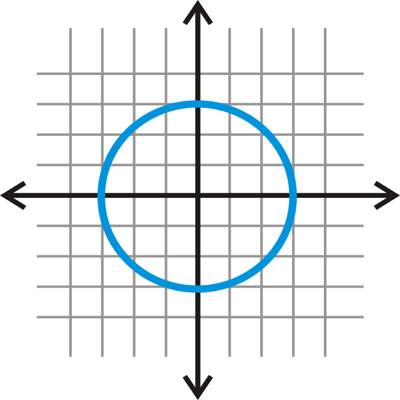Co-ordinates Geometry is a system of geometry where the position of points on the plane is described using an ordered pair of numbers. Straight lines in coordinate geometry are the same idea as in regular geometry, except that they are drawn on a coordinate plane and we can do more with them.

A circle is a round plane figure whose boundary (the circumference) consists of points equidistant from a fixed point (the center).Circle in Co-Ordinate Geometry

(i) The equation of the circle having to center at the origin and radius a unit is x2 + y2 = a2 . . . (1)

The parametric equation of the circle (1) is x = a cos θ, y = a sin θ, θ being the parameter.

(ii) The equation of the circle having to center at (α, β) and radius a unit is (x – α)2 + (y – β)2 = a2.

(iii) The equation of the circle in general form is x2 + y2 + 2gx + 2fy + c = 0 The centre of this circle is at (-g, -f) and radius = √(g2 + f2 – c)

(iv) The equation ax2 + 2hxy + by2 + 2gx + 2fy + c = 0 represents a circle if a = b (≠ 0) and h = 0.

(v) The equation of a circle concentric with the circle x2 + y2 + 2gx + 2fy + c = 0 is x2 + y2 + 2gx + 2fy + k = 0 where k is an arbitrary constant.

(vi) If C1 = x2 + y2 + 2g1x + 2f1y + c1 = 0

and C2 = x2 + y2 + 2g2x + 2f2y + c2 = 0 then

(a) the equation of the circle passing through the points of intersection of C1 and C2 is C1 + kC2 = 0 (k ≠ 1);

(b) the equation of the common chord of C1 and C2 is C1 – C2 = 0.

(vii) The equation of the circle with the given points (x1, y1) and (x2, y2) as the ends of a diameter is (x – x1) (x – x2) + (y – y1) (y – y2) = 0.

(viii) The point (x1, y1) lies outside, on or inside the circle x2 + y2 + 2gx + 2fy + c = 0 according as x12 + y12 + 2gx1 + 2fy1 + c > , = or < 0.

Information Source: